SSC BOARD PAPERS IMPORTANT TOPICS COVERED FOR BOARD EXAM 2024

### Question 19. Balance the following equation stepwise. Ag(s) + HCl(aq) → AgCl + H2

Question 19.

Balance the following equation stepwise.

Ag(s) + HCl(aq) → AgCl + H2

Balanced equation: 2Ag + 2HCl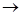2AgCl + H2

Explanation:

⇒ Step 1: Write the given unbalanced equation

Ag + HClAgCl + H2

⇒ Step 2: Compare the number of atoms of reactants with the number of atoms of products.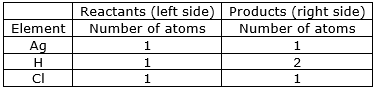⇒ Step 3: Now, First we consider the element having unequal no. of atoms on both sides. Thus, let us consider hydrogen atom. If we multiply 2 in the reactant (in HCl), we will get the equal number of atoms as in product (H2)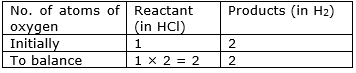⇒ Step 4: Write the resulting equation:

Ag + 2HCl → AgCl + H2

⇒ Step 5: Now check whether the equation is balanced or not by comparing the atoms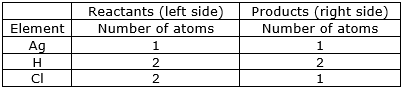We find that the equation is not balanced yet. As the number of

chlorine atom is unequal on the two sides.

First balance the chlorine number.

⇒ Step 6: Now, let us consider chlorine atom. If we multiply 2 in the product (in AgCl), we will get the equal number of atoms as in reactant (in HCl)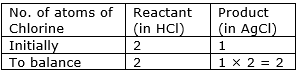⇒ Step 7: Write the resulting equation:

Ag + 2HCl2AgCl + H2

⇒ Step 8: Now check whether the equation is balanced or not by comparing the atoms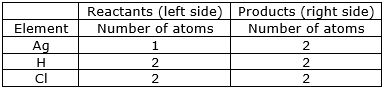We find that the equation is not balanced yet. As the number of

silver atom is unequal on the two sides.

⇒ Step 9: Now, let us consider silver atom. If we multiply 2 in the reactant (Ag), we will get the equal number of atoms as in product (AgCl)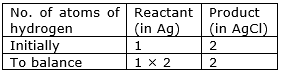⇒ Step 10: Write the resulting equation:

2Ag + 2HCl2AgCl + H2

⇒ Step 11: Now, compare the atoms of both the sides of all the elements. Write down the final balanced equation:

2Ag + 2HCl2AgCl + H2